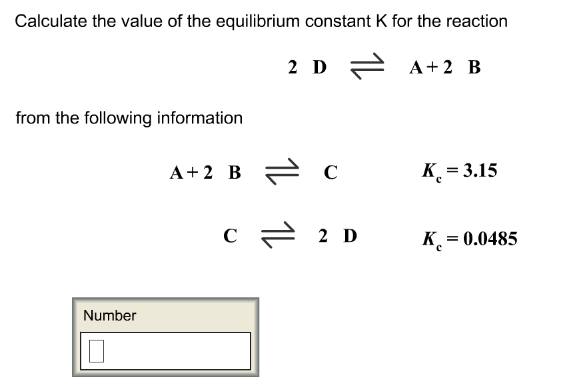Chemistry Practice Problems Equilibrium Expressions Practice Problems Solution: Calculate the value of the equilibrium constant K ...

# Solution: Calculate the value of the equilibrium constant K for the reaction 2D ⇌ A + 2B from the following information A + 2B ⇌ C             Kc = 3.15C ⇌ 2D                   Kc = 0.0485

###### Problem

Calculate the value of the equilibrium constant K for the reaction

2D ⇌ A + 2B

from the following information

A + 2B ⇌ C             Kc = 3.15

C ⇌ 2D                   Kc = 0.0485View Complete Written Solution

Equilibrium Expressions

Equilibrium Expressions

#### Q. Part 1 (1 point) Do not add states of matter to the equilibrium expressions.Write the equilibrium constant expression for the following reaction: N2O ...

Solved • Thu Oct 04 2018 13:18:30 GMT-0400 (EDT)

Equilibrium Expressions

#### Q. At 2000 K the partial pressures of an equilibrium mixture of H2S, H2, and S are 0.015, 0.041, and 0.025 atm, respectively. Calculate the value of the ...

Solved • Tue Sep 18 2018 16:33:17 GMT-0400 (EDT)

Equilibrium Expressions

#### Q. The reaction 2PH3 (g) + As2 (g) ⇌ 2AsH3 (g) + P2 (g) has Kp = 2.9 x 10-5 at 873 K. At the same temperature, what is Kp for each of the following react...

Solved • Mon Sep 17 2018 19:25:57 GMT-0400 (EDT)

Equilibrium Expressions

#### Q. The equilibrium constant, Kc, is calculated using molar concentrations. For gaseous reactions another form of the equilibrium constant, Kp, is calcula...

Solved • Mon Sep 17 2018 19:05:46 GMT-0400 (EDT)